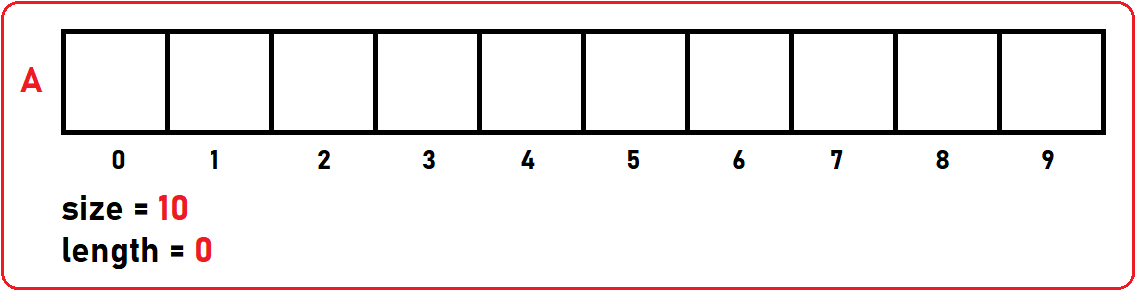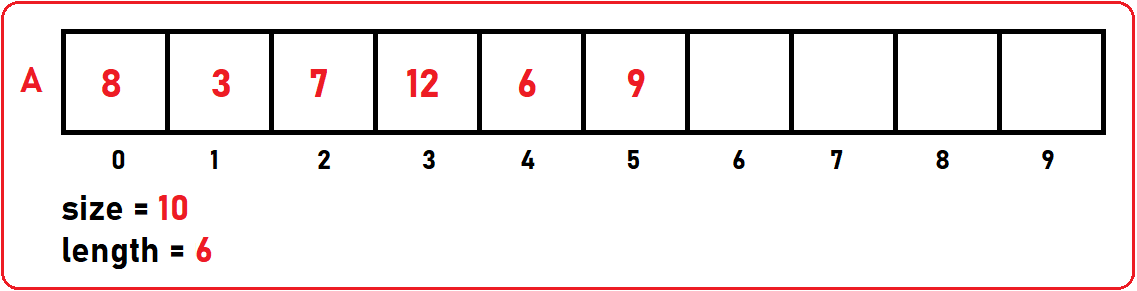# Display Append and Insert Elements in an Array

## Display Append and Insert Elements in an Array using C and C++ Language

In this article, I am going to discuss Display Append and Insert Elements in an Array using C and C++ Language i.e. 3 array operations i.e. Display(), Append(n), and Insert(index, n) with Examples. Please read our previous article, where we discussed Array as ADT (Abstract Data Type).

##### Operations on Arrays

First, we created an array of size 10 and length 0 as there is no element in the array. For better understanding, please have a look at the below image.Then, we fill this array with some elements i.e., 8, 3, 7, 12, 6, 9. Now, our filled Array will look like below. As we insert some elements, we have to increment length also so that it will show the total number of elements present in our array. So, here the length will 6. For better understanding, please have a look at the below image.Now, let’s see all 3 functions one by one.

##### Display Operation on Array –

This function will display each and every element on the screen. So here we are targeting every element present in an array. For this, we are using for loop here. Now the point is the array is of size 10 and we have only 6 elements, so what we have to do here, is just traverse through the array till index of [6-1] because the rest of the array is empty. For better understanding, please have a look at the following code.Now, our next operation is Append ().

##### Add (n) / Append (n) Operation in Array–

Append (n) will add the given element to the end of the given array. The following is our array:In the above array, I want to append 10 to the next to the last element in the array. Here I want to append at the index of arr[length] = n. As length is the position of the last element + 1 because array indexing starts from 0. For better understanding, please have a look at the following code.The time complexity for this operation is O (1) which is constant. Now, our next operation is Insert ().

##### Insert (index, n) Operation in Array–

In this function, we will pass an element and an index position where we want to insert any particular element in our array. For this, we have to check if our array has space for that element or not. So first we check for if (size > length), in our case, yes, so then we will perform shifting of elements. To insert at any particular index, first, we have to shift all the elements to the right side so that there will be space at that index for our new element. Let’s take the example of Insert (4, 14). In the below image we shift 10 from index 6 to 7.Then we are shifting 9 from index 5 to 6.Then we are shifting 6 from index 4 to 5Here finally, all elements have shifted and at the 4th index, we just insert our element.###### Let’s have a look at the code:##### The Complete C Code:
```#include <stdio.h>
#include <stdlib.h>

struct Array {
int* A;
int size;
int length;
};

void Display(struct Array arr) {
printf("\nElements are\n");
for (int i = 0; i < arr.length; i++)
printf("%d ", arr.A[i]);
}

void Append(struct Array* arr, int x) {
if (arr->length < arr->size)
arr->A[arr->length++] = x;
}

void Insert(struct Array* arr, int index, int x) {
if (index >= 0 && index <= arr->length) {
for (int i = arr->length; i > index; i--) {
arr->A[i] = arr->A[i - 1];
}
arr->A[index] = x;
arr->length++;
}
}

int main() {
struct Array arr;

printf("Enter Size of an Array: ");
scanf("%d", &arr.size);

arr.A = (int*)malloc(sizeof(int) * arr.size);
arr.length = 0;

printf("Enter Number of Elements: ");
scanf("%d", &arr.length);

printf("Enter All Elements: \n");
for (int i = 0; i < arr.length; i++) {
scanf("%d", &arr.A[i]);
}

Append(&arr, 10);
Insert(&arr, 0, 12);
Display(arr);

getchar();
}
```
###### Output:##### The Complete C++ Code:
```#include <iostream>
#include <conio.h>
using namespace std;

struct Array {
int* A;
int size;
int length;
};

void Display(struct Array arr) {
cout << "\nElements are\n";
for (int i = 0; i < arr.length; i++)
cout << arr.A[i] << " ";
}

void Append(struct Array* arr, int x) {
if (arr->length < arr->size)
arr->A[arr->length++] = x;
}

void Insert(struct Array* arr, int index, int x) {
if (index >= 0 && index <= arr->length) {
for (int i = arr->length; i > index; i--) {
arr->A[i] = arr->A[i - 1];
}
arr->A[index] = x;
arr->length++;
}
}

int main() {
struct Array arr;

cout << "Enter Size of an Array: " << endl;
cin >> arr.size;

arr.A = new int[arr.size];
arr.length = 0;

cout << "Enter Number of Elements: " << endl;
cin >> arr.length;

cout << "Enter All Elements: " << endl;
for (int i = 0; i < arr.length; i++) {
cin >> arr.A[i];
cout << " ";
}

Append(&arr, 10);
Insert(&arr, 0, 12);
Display(arr);

getchar();
}
```
###### Output:In the next article, I am going to discuss How to DELETE an Element in an Array with Examples. Here, in this article, I try to explain How to Display Append and Insert Elements in an Array using C and C++ Language with Examples and I hope you enjoy this How to Display Append and Insert Elements in an Array article.